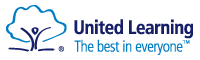# Nucleus STEM Curriculum

### Students within the Nucleus Gifted STEM Stream will cover broadly the same curriculum as the rest of their year group with some notable exceptions; Biology, Chemistry, Physics, Mathematics and Computer Science.

The amount of curriculum time for these subjects varies, with a reduction in curriculum time for certain other subjects to allow greater access to the STEM subjects. All Nucleus students will be targeted at grade 9 in their GCSEs in the Sciences, Mathematics and Computer Science.

Nucleus students will have access to small group peripatetic Music lessons, learning to play an instrument of their choosing.  The cost of this is covered by Nucleus STEM Stream.

### Nucleus STEM Mathematics Curriculum

Within the Nucleus STEM Mathematics Curriculum, students will study Mathematics at an accelerated rate with the aim of completing the Key Stage 3 content within 2 years.

In addition to the 480 minutes of curriculum time per fortnight, students are expected to attend a once weekly session, making use of the online platform Hegarty Maths as part of their co-curricular programme.

Year 7 Nucleus Mathematics Topic Summary

### Term 1

Number and Shape

Basic operations

Factors and multiples

Area and perimeter

### Term 2

Geometry and Shape

Angles

Coordinates

Transformations of shapes

### Term 3

Fractions, Decimals and Percentages

Fraction operations

Converting between fractions, decimals and percentages

Percentages

### Term 4

Algebra

Introduction to algebra

Expanding and factorising

Linear equations

### Term 5

Shape

Volume and surface area of shapes

Pythagoras

### Term 6

Statistics

Calculating averages

Representing and interpreting data

Year 8 Nucleus Mathematics Topic Summary

### Term 1

Number and Measure

Indices

Standard Form

Measurements

### Term 2

Algebra

Linear and quadratic equations

Simultaneous equations

Straight line graphs

### Term 3

Trigonometry

Introduction to Trigonometry

3D Pythagoras

### Term 4

Ratio and Geometry

Ratio

Algebraic proportion

Circle theorems

Number

Percentages

Bounds

Data project

### Term 6

Algebra

Rearranging formulae

Complex graphs

Year 9 Nucleus Mathematics Topic Summary

### Term 1

Geometry and Statistics

Vectors

Probability

Representing data

### Term 2

Number and Geometry

Percentages

Recurring decimals

Trigonometry

### Term 3

Algebra

Expanding and factorising

Algebraic fractions

Graphs

### Term 4

Algebra

Simultaneous equations

Graphs

Inequalities

### Term 5

Sequences and Geometry

Sequences

Iteration

Constructions and loci

### Term 6

Algebra and Geometry

Functions

Graph transforations

Circle theorems

Year 10 Nucleus Mathematics Topic Summary

### Term 1

Algebra, Statistics and Geometry

Complex graphs

Representing and interpreting data

Constructions and loci

### Term 2

Algebra and Geometry

Algebraic proof

Circle theorems

Plans and evaluations

### Term 3

Shape and Geometry

Similar shapes

Congruence

Bearings

### Term 4

Graphs and Trigonometry

Real-life graphs

Graph transformations

Further Trigonometry

Algebra

Functions

Sequences

Surds

### Term 6

Algebra